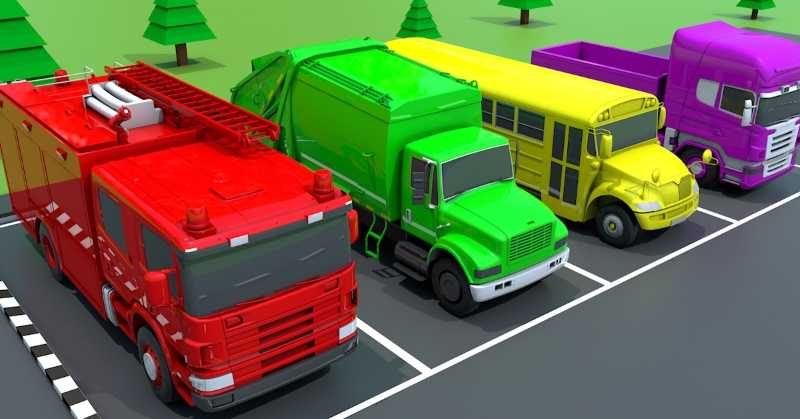Let's do some simple calculations.Which one is wider?Let's count ice creams and sandwiches together!Do you like crayons? Let's learn colors with crayons.Which one is the animal we want?Let's do some simple calculations.Can you tell the order of these fruits?Can you count these strawberries and apples?Let's count chickens together!Can you count these melons?Let's count these celery together!Can you count these onions and garlics?Can you count these food?Let's count these plums together!Let's do some simple counting.Can you count these cupcakes?What is the missing letter in this word?Let's count these dragon fruits together!Can you count these potatoes please?Can you count these grapes?